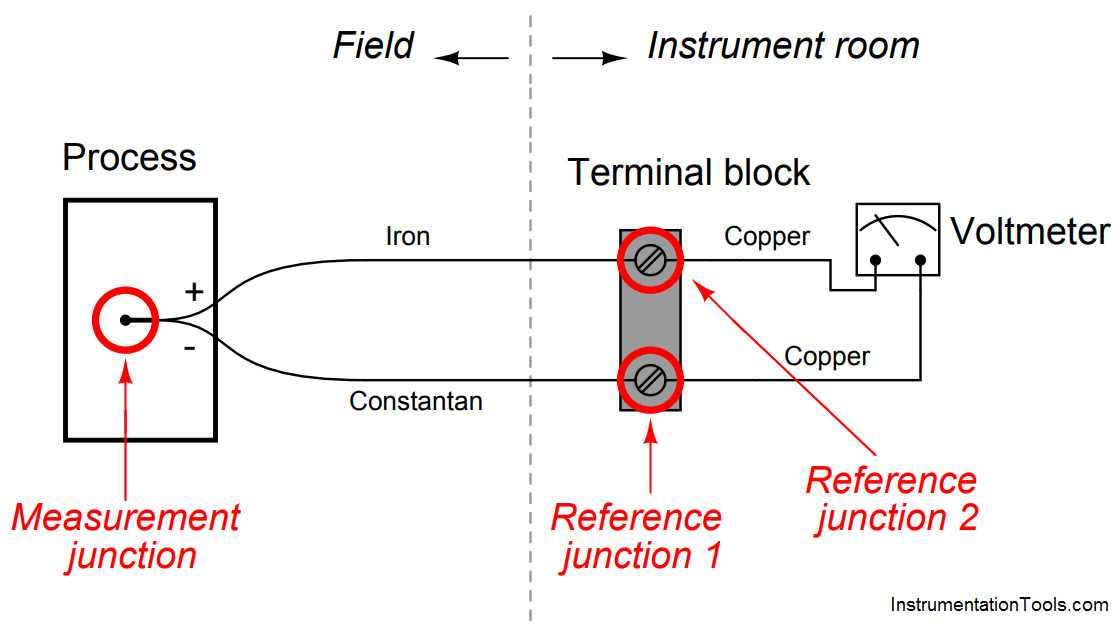# Thermocouple Terminal block Questions

When a type “T” thermocouple (copper/constantan) is connected to a voltmeter made of copper wires, two active junctions are formed: one at the point of measurement (the measurement junction) and one at a terminal near the voltmeter (the reference junction). The copper-to-copper junction at the top screw of the terminal block is of no consequence because it is a junction of identical metals, and as such generates no thermoelectric voltage:The amount of voltage sensed by the voltmeter in this thermocouple circuit is equal to the difference in voltages produced by the measurement and reference junctions:

Emeter = Emeas − Eref

Now consider a type “J” thermocouple connected to a copper-wire voltmeter. Here we see there are not two but three active junctions of dissimilar metals:Upon first observation it would appear this circuit is more complicated than the type “T” thermocouple circuit, owing to the existence of the additional dissimilar-metal junction. However, a principle called the Law of Intermediate Metals allows us to consider the two reference junctions (iron-copper and constantancopper) as electrically equivalent to a single reference junction of iron-constantan, such that the type “J” thermocouple circuit becomes just as simple as the type “T” circuit with one measurement junction and one reference junction in opposition to each other.

Explain what the Law of Intermediate Metals is, and how it may be used to simplify the two active reference junctions of the type “J” circuit.

Also, explain why it is important that the terminal block be isothermal in nature.

The Law of Intermediate Metals tells us that a series chain of dissimilar metal junctions at the same temperature are electrically equivalent to a single junction comprised of the outer two metals (ignoring all the intermediate metal types between) at that temperature.

Thus, the iron-copper-constantan reference junction pair is electrically equivalent to a single iron-constantan reference junction, so long as both screw terminals are at the same temperature (isothermal). This principle renders the meter’s metal type irrelevant.

Don't Miss Our Updates
Be the first to get exclusive content straight to your email.
We promise not to spam you. You can unsubscribe at any time.
1.